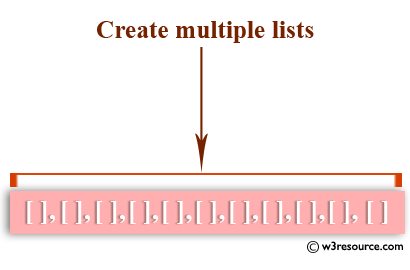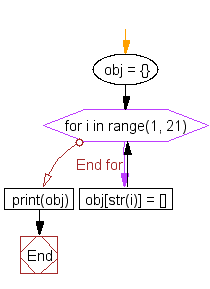﻿ Python: Create multiple lists - w3resource# Python: Create multiple lists

## Python List: Exercise - 41 with Solution

Write a Python program to create multiple lists.Sample Solution:-

Python Code:

``````obj = {}
for i in range(1, 21):
obj[str(i)] = []
print(obj)
```
```

Sample Output:

```{'1': [], '8': [], '14': [], '5': [], '17': [], '9': [], '2': [], '7': [], '16': [], '19': [], '4': [], '18':
[], '13': [], '3': [], '15': [], '11': [], '20': [], '6': [], '12': [], '10': []}
```

Flowchart:## Visualize Python code execution:

The following tool visualize what the computer is doing step-by-step as it executes the said program:

Python Code Editor:

Have another way to solve this solution? Contribute your code (and comments) through Disqus.

What is the difficulty level of this exercise?

Test your Python skills with w3resource's quiz

﻿

## Python: Tips of the Day

```print(2_000_000)
```2000000